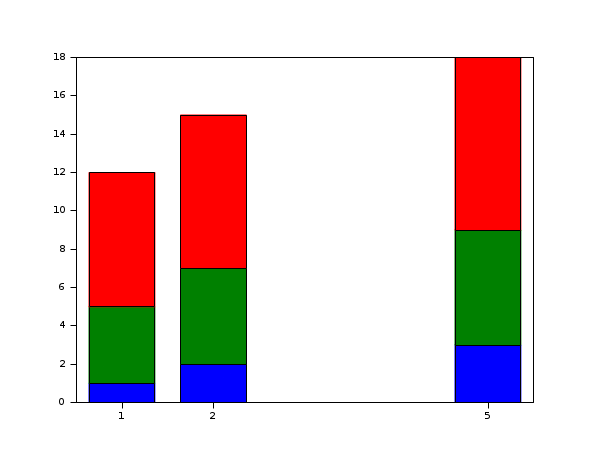Scilab Home page | Wiki | Bug tracker | Forge | Mailing list archives | ATOMS | File exchange
Scilab 5.5.0
Change language to: English - Français - Português - 日本語 -

Please note that the recommended version of Scilab is 6.0.2. This page might be outdated.
See the recommended documentation of this function

# bar

bar histogram

### Calling Sequence

```bar(y)
bar(x,y)
bar([h],x,y [,width [,color [,style]]])```

### Arguments

h

an axes handle, (default: h=gca() ).

y

a real scalar, vector of size N, or a matrice N*M.

x

a real scalar or a vector of size N, (default: if y is a vector then x is a vector and x length equals to y length. If y is a matrix then x is a vector and x length equals to the lines number of y.

width

(optional), a real scalar, defines the width (a percentage of the available room) for the bar (default: 0.8, i.e 80%).

color

(optional), a string (default: 'blue'), specifying the inside color bar.

style:

a string, 'grouped' or 'stacked' (default: 'grouped').

### Description

`bar(y,...) :` if y is a vector then bar function draws a polyline which has the `polyline_style` type 6. If y is a vector, bar draws vector y versus vector 1:size(y,'*') . If y is a matrix N*M, bar draws M polylines (type 6), each polyline corresponds to a column of y versus vector x=1:size(y,1).

`bar(x,y,...) :` if y is a vector then bar function draws a polyline which has the `polyline_style` type 6, where x length = y length.If y is a matrix NxM then bar function draws M polylines which have the type 6. Each polyline corresponds to a column of y versus vector x.

`bar(h,...) :` defines the current axes where the drawing is performed.

`bar(...,width,...) :` defines the width of the bar(s) in percentage (generally: 0<width<=1).

`bar(...,style,...) :` defines how the bar is drawn. If y is a matrix N*M (so M polylines of type 6) then there are two ways to draw the M bars. the style option = 'grouped' allows to center the M polylines versus each components of x, and the style option 'stacked' allows to stack them.

`bar(...,color,...) :`defines the bar color. Bar functions uses the same colormap than in the `plot` function.

If there are several bar calls, the `barhomogenize` function allows to homegenize the width and style of all bars (i.e polylines of type 6) included in the current working axes.

### Examples

```// First example: draw a bar (i.e a polyline with polyline_style type =6) with
// width=0.5 and color='yellow' and default style='grouped', x=1:length(y).
scf(0);
y=[1 -3 5];
bar(y,0.5,'yellow');``````// Second example: draw 3 bars (i.e 3 polylines with polyline_style type =6),default style='grouped'
scf(1);
x=[1 2 5];
y=[1  -5 6;3 -2 7;4  -3 8];
bar(x,y);``````// Third example : style='stacked'.
scf(2);
x=[1 2 5];
y=[1 4 7;2 5 8;3 6  9];
bar(x,y,'stacked');``````// Fourth example: width=0.2;color='green'; default style='grouped'
scf(3);
x=[1 2 5];
y=[1 4 7;2 5 8;3 6 9];
bar(x,y,0.2,'green');```• barh — horizontal display of bar histogram
• barhomogenize — homogenize all the bars included in the current working axes
• plot — 2D plot
• histplot — plot a histogram
• polyline_properties — description of the Polyline entity properties